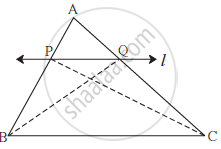# Basic Proportionality Theorem (Thales Theorem)

#### Topics

• Theorem: If a line parallel to a side of a triangle intersects the remaining sides in two distinct points, then the line divides the sides in the same proportion.

## Notes

Theorem: If a line parallel to a side of a triangle intersects the remaining sides in two distinct points, then the line divides the sides in the same proportion.

Given: In D ABC line l || line BC and line l intersects AB and AC in point P and Q respectively

To prove: "AP"/"PB"="AQ"/"QC"

Construction: Draw seg PC and seg BQProof: Δ APQ and Δ PQB have equal heights.

therefore (A(triangle APQ))/(A(triangle PQB))="AP"/"PB" .....................(I) (areas proportionate to bases)

therefore (A(triangle APQ))/(A(triangle PQC))="AQ"/"QC"................... (II) (areas proportionate to bases)

seg PQ is a common to base of Δ PQB and ΔPQC. seg PQ || seg BC,

hence Δ PQB and Δ PQC have equal heights.

A(triangle PQB)=A(triangle PQC) ................(III)

(A(triangle APQ))/(A(triangle PQB))=(A(triangle APQ))/(A(triangle PQC))  .......... [from (I), (II) and (III)]

therefore "AP"/"PB"="AQ"/"QC" .......... [from (I) and (II)]

If you would like to contribute notes or other learning material, please submit them using the button below.

### Shaalaa.com

Triangles part 6 (Example Thales theorem) [00:11:30]
S
0%